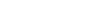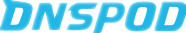• 注册时，本站名称为：RGB空格3S博客，注意中间的空格。
• 2017.2.14今天收到45条恶意评论（全是外文），故评论时请填写必要信息，匿名评论全部拉黑,迫不得已而为之
• 2017.1.27,2017年春节及至，我谨代表本人祝大家新春快乐，本人年终总结文章请访问：2016年终总结
• 为防止恶意转载，本站全面禁止复制，并添加图片水印：RGB 3S博客www.ixxin.cn。
• 本站正式更名为RGB 3S博客，本站将撤消所有非3S内容，其将转移到新博客江湖时代

# C++生成一个随机二维数组并作为参数返回

7245次浏览
[隐藏]

## 代码

```/*

*/

#include<iostream>
#include<stdlib.h>
#include<ctime>
using namespace std;

int **random(int n,int m)
{
int t;
int **data;
t = time(0);
srand(t);
data=(int **)malloc(n*sizeof(int *));
for (int i=0;i<n;i++)
data[i]=(int *)malloc(2*sizeof(int));
for(int i = 0;i<n;i++)
{
for(int j = 0;j<m;j++)
{
data[i][j] = rand()%10;
}
}
return data;
}

int visit(int **data,int n,int m)
{
for(int i = 0;i<n;i++)
{
for(int j = 0;j<m;j++)
cout<<data[i][j]<<" ";
cout<<endl;
}
return 0;

}

int main()
{

int n,m;
cout<<"请输入行数,列数"<<endl;
cin>>n;
cin>>m;
int **data = random(n,m);
visit(data,n,m);
free(data);
system("pause");
return 0;
}
```## 后语

xxin blog , 版权所有丨如未注明 , 均为原创丨本网站采用BY-NC-SA协议进行授权 , 转载请注明C++生成一个随机二维数组并作为参数返回• 版权声明

本站的文章和资源来自互联网或者站长
的原创，按照 CC BY -NC -SA 4.0 CN
协议发布和共享，转载或引用本站文章
应遵循相同协议。如果有侵犯版权的资
源请尽快联系站长，我们会在24h内删
除有争议的资源。
• 网站驱动

• GIT主题
•••• 友情链接

• 支持站长